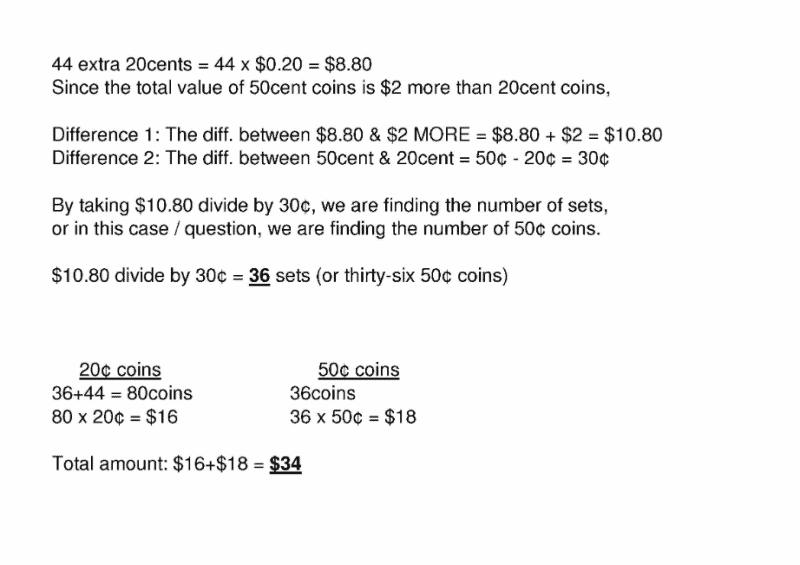Question

pls help me solve

rodney has 44 more 20cents coins than 50cents coins in his coin box. the total value of the 50cents coins is 2\$ more than the total value of the 20cents coins.

a) how many 50cents coins are there in his coin box

b)how much does rodney haveCurioustudent’s method is faster and more straight forward.

If you could understand his/her method, then using his/hers would be better.

Hope this helps.

your one is much easier to use and to understand. Thanks!

0 Replies 0 Likes

Let x be the amount of 50 cents coins
(44+x)0.2=0.5x-2

8.8+0.2x=0.5x-2

10.8=0.3x

x=36

a)36

(44+36)0.2+(36)0.5=16+18=34
b)\$34